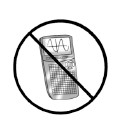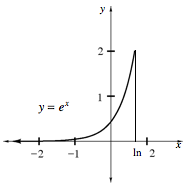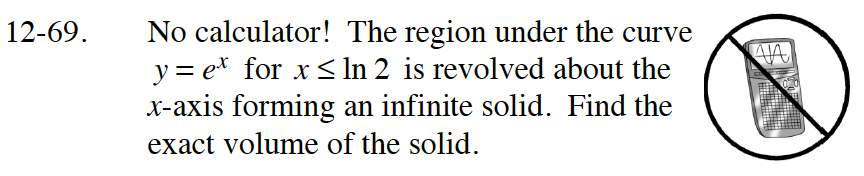### Home > CALC > Chapter 12 > Lesson 12.2.1 > Problem12-69

12-69.

No calculator! The region under the curve y = ex for x ≤ In 2 is revolved about the x-axis forming an infinite solid. Find the exact volume of the solid. Homework Help ✎$\lim_{a\to -\infty}\int_a^{\ln(2)}\pi (e^x)^2dx$